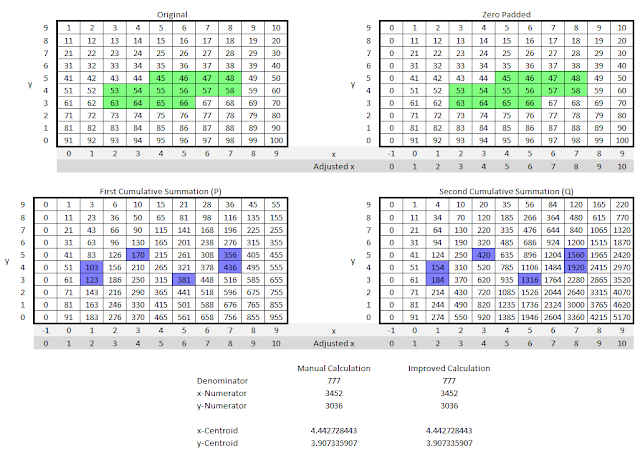## Friday, April 21, 2017

### I hope you find this blog enjoyable and helpful. Support it with a small donation.

A project I'm working on requires the repeated calculation of weighted centroids for arbitrary regions in an image.  Calculation of centroids is fairly straight forward but computationally expensive.  To calculate the x and y coordinates you first need to calculate three other values, the denominator, x-numerator and y-numerator.  The denominator is simply equal to the sum of all the values in the region, the x-numerator is the sum of all the values in the region multiplied by their x coordinate, and the y-numerator is the sum of all the values in the region multiplied by their y coordinate.  From these values the x coordinate of the centroid is equal to the the x-numerator divided by the denominator likewise for the y coordinate of the centroid.

You can see from this description that there are a lot of time consuming multiplication operations to perform for each area that you want to calculate the centroid for.  As it turns out though, you can perform two cumulative summations along the rows of the input data as a first step, store this and then perform the centroid calculation by only accessing values at the boundary of the region. For reasons I'll explain later a column of zeros with x coordinate -1 is added to the start of the data.  A cumulative summation is performed along each row to obtain $P$. A cumulative summation along each row of $P$ is performed to generate $Q$ The following equations describe the process.  Before we get started, the derivation of these  equations can be found in this small article I wrote.Centroid Calculations
It may not be immediately obvious what these mean so I'll give a quick explanation.  First of all each of the above calculations are done row by row and added together. This is what the summation symbol over $y$ represents.  For the denominator the value to be summed is the difference between two values on a row of $P$. For the y-numerator the calculation is the same as the denominator but each row is multiplied by the y coordinate.  The calculation of the x-numerator is a little different. It's similar in that values of $P$ at the boundary are subtracted and multiplied by $x+1$ but the additional step of subtracting the difference of the values of $Q$ at the boundaries is now added.  Maybe an example will help.Example Calculation

In the first data set on the top left an area is marked green.  The centroid of this region is to be found. A column of zeros is added to the start of the data.  This is seen in the data set on the top right.  These are added because when performing the operation in software you end up accessing array outside of their bounds.

Typically when calculating centroids each green cell would have to be accessed for each calculation. However when using the new method described above only the cells in blue are accessed.  You may also notice that the width of these doesn't change the number of operations.  The complexity of the calculation is only dependant on the height of the region.  I've included this spreadsheet for you to play around with and get a better understanding of the process.

One last this to note is that the addition of the column of zeros while maintaining the original $x$ indices is silly as it creates a column with an index of negative one.  This is where the adjusted x index comes in.  Using this and the following adjusted equations allow the calculation to be performed easily on computers.

Let's for example calculate the centroid of the green section on only row 5.  Its $x$ bounds are $x_1=4$ and $x_2=7$, but in the above equations $P$ and $Q$ are accessed via the adjusted $x$ index at 4 and 8 (8 because of +1 on the upper index).  This means the denominator is equal to $(356-170) = 186$, the y-numerator is equal to $5(356-170)=930$, and the x-numerator is equal to $(8 \times 356-4 \times 170)-(1560-420)=1028$.  This leads to centroid coordinates of (5.526, 5). This is what you would expect as it's a single row the y value is obviously 5 and the x value is a little to the right of centre due to the increasing trend in the numbers. The centroid coordinates calculated are given in the original x index to allow accessing the original data.